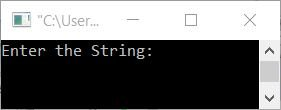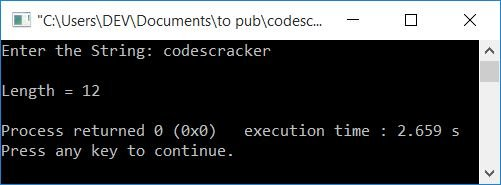# C++ Program to Find the Length of a String

In this article, you will learn how to find and print the length of any given string given by the user at run-time in the C++ language. The program was created with the help of these approaches:

## In C++, find the length of a string without using the strlen() function

To find the length of a string in C++ programming, you have to ask the user to enter the string first. and then find its length as shown in the program given below.

This program finds the length of a string using user-based code. That is, this program does not use library functions or the built-in function strlen().

```#include<iostream>
#include<stdio.h>
using namespace std;
int main()
{
char str;
int len=0, i=0;
cout<<"Enter the String: ";
gets(str);
while(str[i])
{
len++;
i++;
}
cout<<"\nLength = "<<len;
cout<<endl;
return 0;
}```

This program was built and runs under the Code::Blocks IDE. Here is its sample run:Now enter any string, say codes cracker dot com, and press the ENTER key to find and print the length of the given string, as shown here in the following output:Here is another sample run with user input, codescracker:The dry run of the above program with user input, "codescracker" goes like this:

• Initial values, len=0, i=0
• When the user enters the string, say codescracker, as input, then it gets stored in str[] in this way:
• str=c
• str=o
• str=d
• and so on up until
• str=r
• Now the condition of the while loop gets evaluated. That is, the condition str[i] or str or c evaluates to be true. Therefore, program flow goes inside the loop.
• When there are no more character, the condition evaluates to false. That is, its condition evaluates to false at the 12th index.
• Within the loop, the value of len and i are now incremented. As a result, i = 1 and len = 1.
• The program flow goes back and evaluates the condition again. Because the condition str[i] or str again evaluates to be true, the program flow again goes inside the loop.
• This process is repeated until the last character of codescracker is reached.
• And each time the value of len is increased.
• So when the condition of the while loop evaluates to be false, then we'll have a variable, len, that holds the length of the string, or how many characters are available in the string entered by the user at run-time.
• So print the value of len as output, which shows the length of the given string.

## In C++, use the strlen() function to determine the length of a string

Here is another C++ program that also finds and prints the length of a string entered by the user. The only difference with the previous program is that this program uses a built-in or library function of C++ named strlen().

The function strlen() takes a string as its argument and returns its length. This function is defined in the string.h header file.

```#include<iostream>
#include<stdio.h>
#include<string.h>
using namespace std;
int main()
{
char str;
int len=0;
cout<<"Enter the String: ";
gets(str);
len = strlen(str);
cout<<"\nLength = "<<len;
cout<<endl;
return 0;
}```

The output produced by this program is similar to that of previous programs.

## In C++, find the length of a string using a pointer

Let's make the same program, but this time we'll use pointers to find the length of a string.The address of the first character of a string gets initialized to a pointer type variable, say ptr, and using this variable, the length of the string gets calculated.

Note: The & is called as the address of operator. Whereas as the * is called as the value at operator. The ptr++ (char pointer type variable) moves to the next character's address

```#include<iostream>
#include<stdio.h>
using namespace std;
int main()
{
char str, *ptr;
int len=0;
cout<<"Enter the String: ";
gets(str);
ptr = &str;
while(*ptr)
{
len++;
ptr++;
}
cout<<"\nLength = "<<len;
cout<<endl;
return 0;
}```

#### The same program in different languages

C++ Quiz

« Previous Program Next Program »